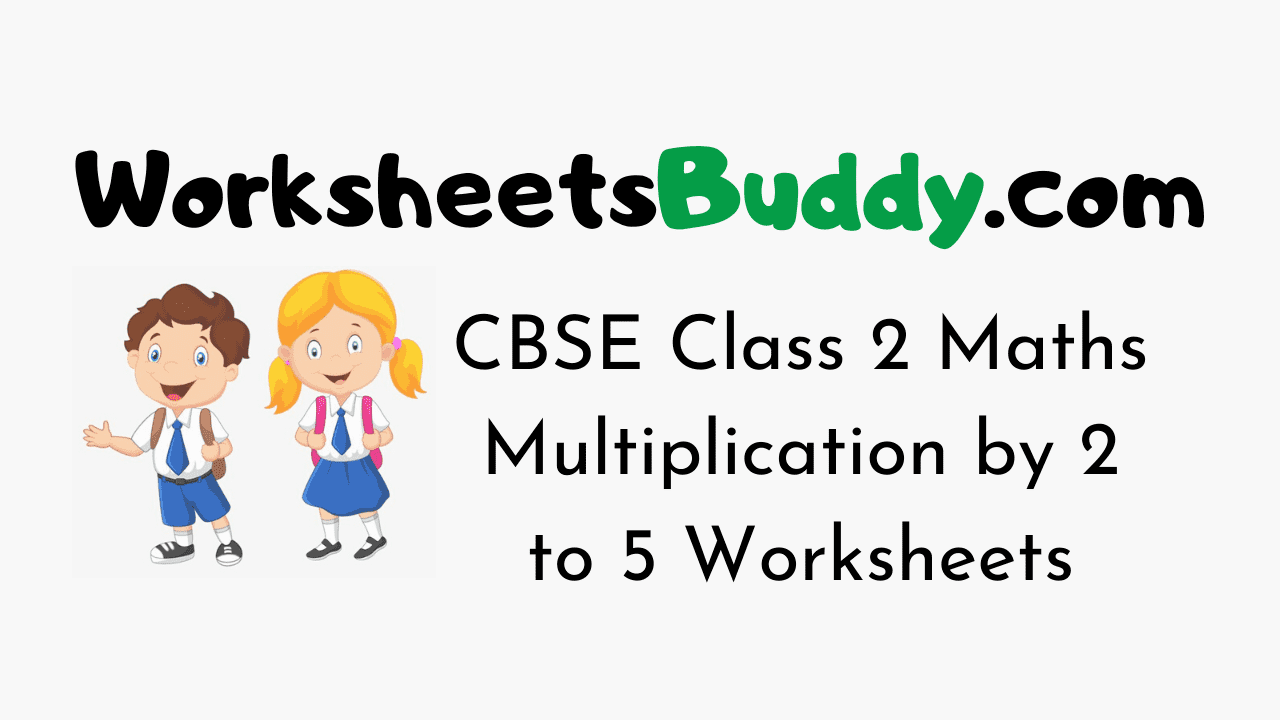# CBSE Class 2 Maths Multiplication by 2 to 5 Worksheets

CBSE Class 2 Maths Multiplication by 2 to 5 Worksheets – Worksheets have become an integral part and are the most engaging study materials for kids these days. The Standard 2 Maths Multiplication by 2 to 5 Worksheets will help your younger one to practice what they have learned in class. Multiplication by 2 to 5 Worksheets for Grade 2 will develop a curiosity in your kid to learn.

Maths Worksheets for Class 2 are a perfect combination of fun and learning. Find the most interesting and relevant puzzle activities for your kids here. In the Mathematics Grade 2 Multiplication by 2 to 5 Worksheets Puzzles will be in the form of crosswords, true or false, pictures, mazes, fill-ups, MCQs, objectives, questions and answers, and so on.

## Download Free Printable Class 2 Maths Multiplication by 2 to 5 Worksheets

Mathematics Worksheets for Class 2 on Multiplication by 2 to 5 will help your kid remember what he/ she learned in school for a long time. Your Grade 2 kid will love to solve these engaging exercises and interesting collections of Puzzles.

### CBSE Class 2 Maths Multiplication by 2 to 5 Worksheets

1. Multiply : 9 × 2

2. 21*21
A) 221
B) 441
C) 521
D) 653

3. Multiply : 6 × 2

4. Multiply : 10 × 3

5. Multiply : 5 × 3

6. Multiply : 5 × 2

7. Multiply : 2 × 3

8. Multiply : 5 × 6

9. Multiply : 3 × 3

10. Multiply : 8 × 2

11. Multiply : 7 × 4

12. Multiply : 7 × 3

13. Multiply : 9 × 3

14. Multiply : 8 × 5

15. Multiply : 6 × 3

16. Multiply : 2 × 4

17. Multiply : 4 × 3

18. Multiply : 4 × 5

19. Multiply : 5 × 5

20. Multiply : 10 × 4

### CBSE Class 2 Maths Worksheet Answers

1. 18
2. Option B
3. 12
4. 30
5. 15
6. 10
7. 6
8. 30
9. 9
10. 16
11. 28
12. 21
13. 27
14. 40
15. 18
16. 8
17. 12
18. 20
19. 25
20. 40### FAQs on Maths Multiplication by 2 to 5 Class 2 Worksheets

1. How does a child learn Mathematics Multiplication by 2 to 5 at Class 2 Level?

A child can learn Maths Multiplication by 2 to 5 at Class 2 Level through the worksheets prevailing on our page.

2. What are the topics in Maths Class 2?

Mathematics Class 2 will have topics like Multiplication by 2 to 5 etc.

3. Where can I get Mathematics Worksheets for Class 2 Multiplication by 2 to 5 for free?

You can get Maths Multiplication by 2 to 5 Worksheets Class 2 free of cost from our Worksheetsbuddy.com page. You can use them for your kid to get practice.

4. Are the CBSE Class 2 Multiplication by 2 to 5 Worksheets over here printable?

Yes, the CBSE Class 2 Multiplication by 2 to 5 Worksheets here are printable and you can download them easily.

Conclusion

We Hope the information prevailing on our page regarding CBSE Class 2 Mathematics Multiplication by 2 to 5 Worksheets has been useful to you. For more info feel free to reach us and we will assist you at the easiest possible. Keep in touch with our site for more information on Class Wise Worksheets for various subjects.